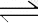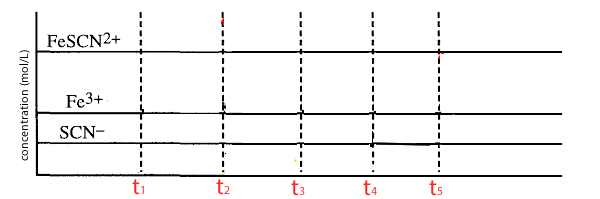Equilibrium activity. Fe3+(aq) (Yellow) + SCN-(aq) (Clear)FeSCN2+(aq) (Red) ∆H = -ve This activity will investigate the effect of the equilibrium position, of the reaction listed above, when the concentration of one of the reactants is changed. The Fe3+ ion appears yellow in high concentrations but clear in low concentrations. SCN- ions are colourless while the FeSCN- ion ranges from brown (low concentrations) to deep red in high concentrations. Such a reaction gives us an opportunity to visualise how the equilibrium shifts when a reactant concentration is changed. Look at the video of the addition of Fe(III) ions and explain how the equilibrium shifts. Look at the video of the addition of SCN- ions and explain how the equilibrium shifts. Look at the video of the removal of Fe(III) ions by the addition of NaF according to the reaction below. Fe3+ (aq) + 6F- (aq) -> FeF63-(aq) Explain how the equilibrium shifts. Why does the solution around where the crystals of NaF are added go clear? Below is a concentration vs time set of axes. Plot what you think is happening as we: T1 = add Fe(III) ions. The system reaches equilibrium before T2 T2 = Add SCN- ions. The system reaches equilibrium before T3 T3 = Remove Fe(III) ions. The system reaches equilibrium before T4 Also include : T4 = a small amount of distilled water is added. The system reaches equilibrium before T5 T5 = the equilibrium mixture is cooled.Solution